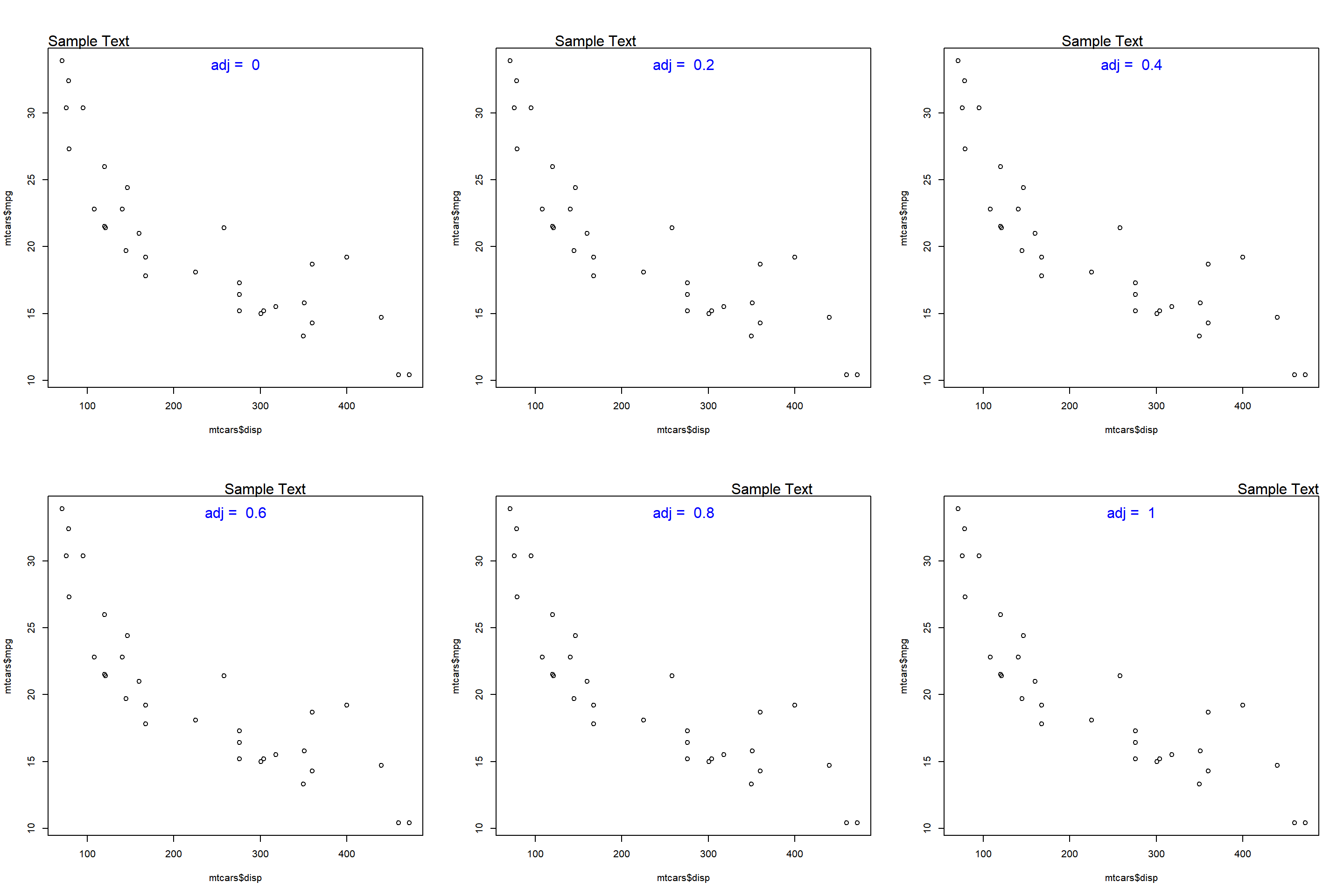# Chapter 9 Text Annotations

## 9.1 Introduction

In this chapter, we will learn to add text annotations. There are occassions when you want to display additional information in a plot. This is usually achieved by adding text either inside the plot or on the margins. For example, you might want to label a line/bar or add formulas to better communicate what is shown in the plot. The idea is to use the available space within/outside the plot to provide additional information that can be useful to the end users. We will learn to add text inside as well as on the margins of the plot. This is accomplished using the following two functions:

• `text()` : add text inside the plot
• `mtext()` : add text on the margins of the plot

## 9.2 Syntax

Let us take a quick look at the syntax of both the functions:

``````text(x, y = NULL, labels = seq_along(x\$x), adj = NULL,
pos = NULL, offset = 0.5, vfont = NULL,
cex = 1, col = NULL, font = NULL, ...)

mtext(text, side = 3, line = 0, outer = FALSE, at = NA,
adj = NA, padj = NA, cex = NA, col = NA, font = NA, ...)``````

## 9.3 Text Inside the Plot

To add text inside a plot, the following arguments must be supplied to the `text()` function:

• `labels` : the text to be displayed
• `x` : x axis coordinate
• `y` : y axis coordinate

Below is a simple example:

``````plot(mtcars\$disp, mtcars\$mpg)
text(x = 340, y = 30, labels = 'Sample Text')``````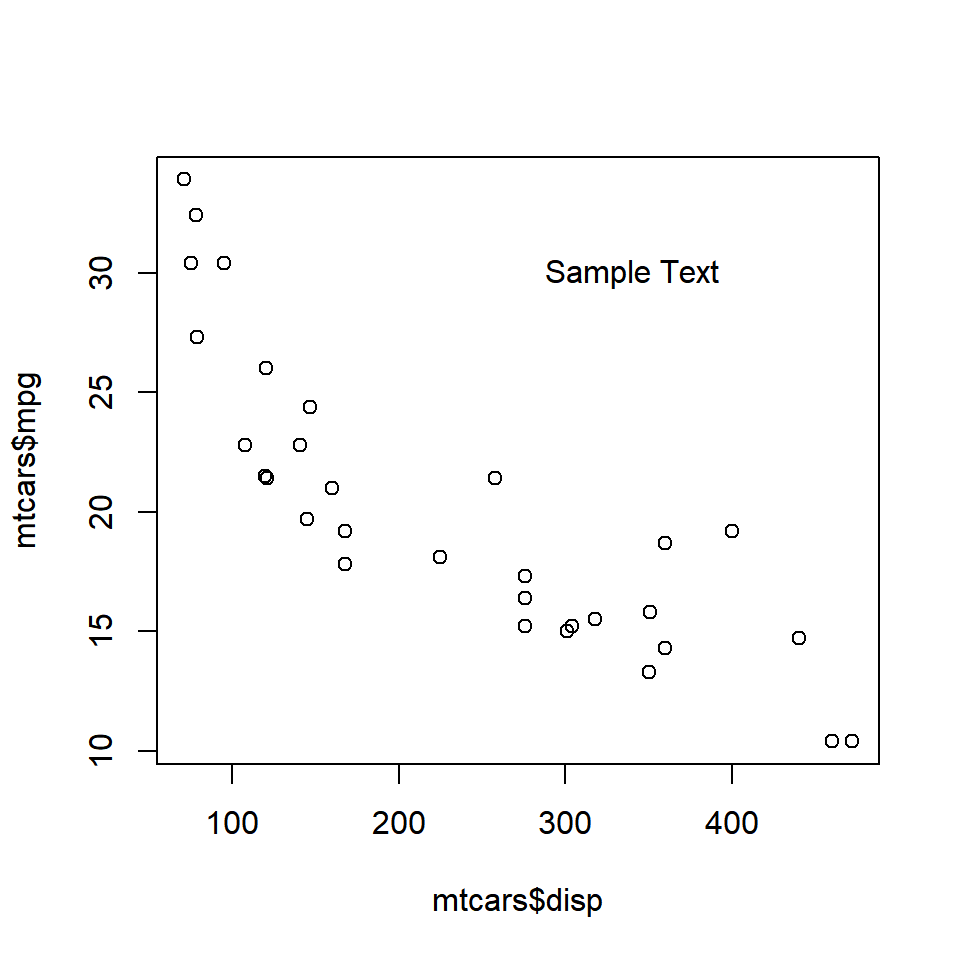The text appears at the coordinates (340, 30). Ensure that the text is enclosed in single/double quotes and the coordinates provided are within the range of the X and Y axis variables.

## 9.4 Color

The color of the text can be modified using the `col` argument in the `text()` function.

``````plot(mtcars\$disp, mtcars\$mpg)
text(x = 340, y = 30, labels = 'Sample Text', col = 'red')``````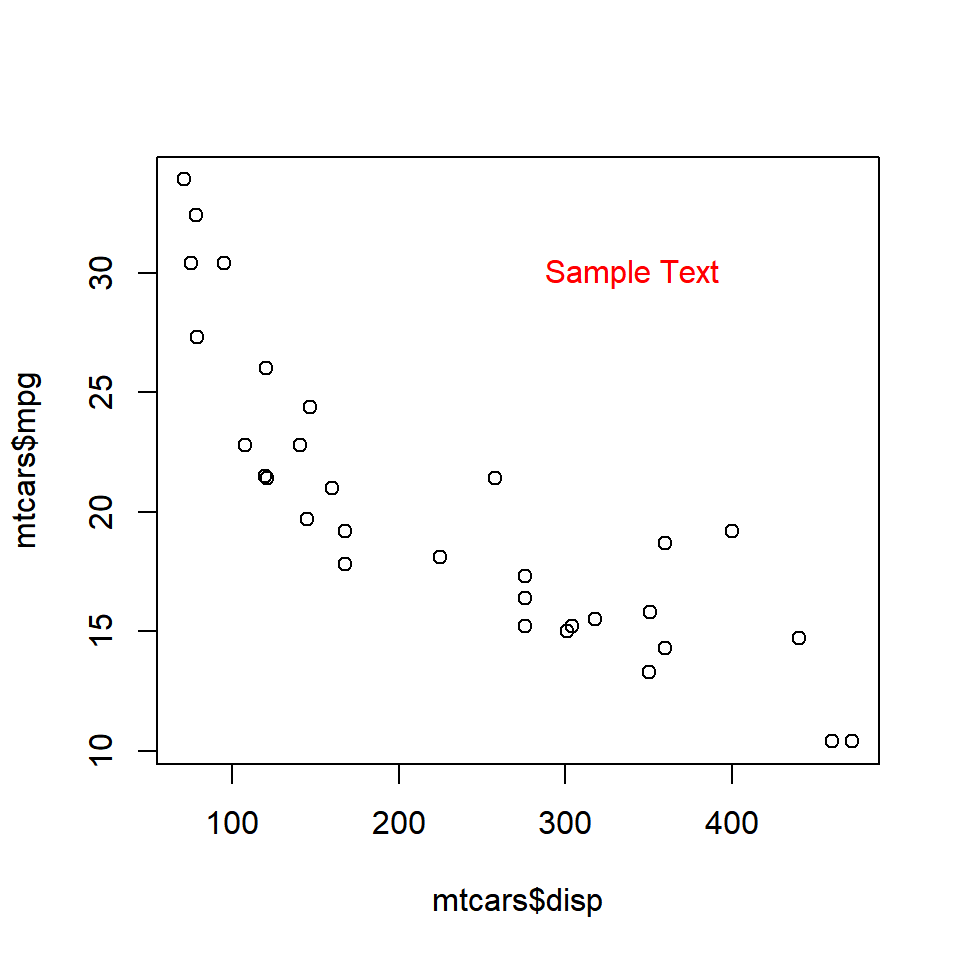The below plot depicts the appearance of the text for different values of the `col` argument: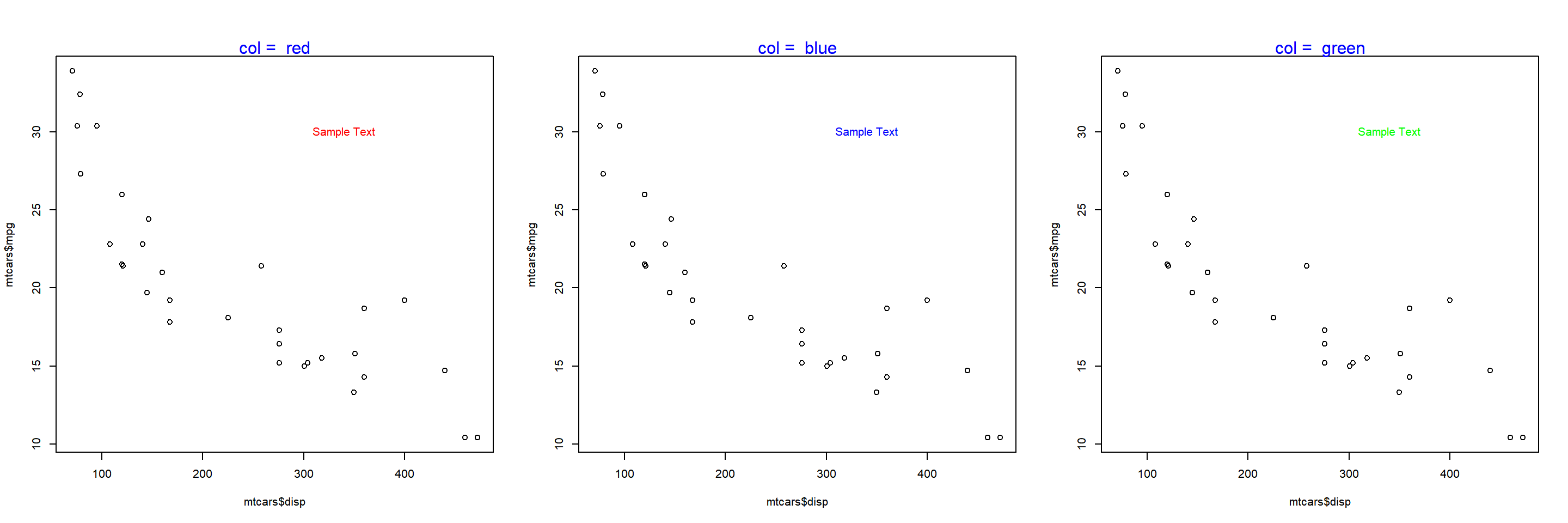## 9.5 Font

The font of the text can be modified using the `font` argument in the `text()` function.

``````plot(mtcars\$disp, mtcars\$mpg)
text(x = 340, y = 30, labels = 'Sample Text', col = 'red', font = 2)``````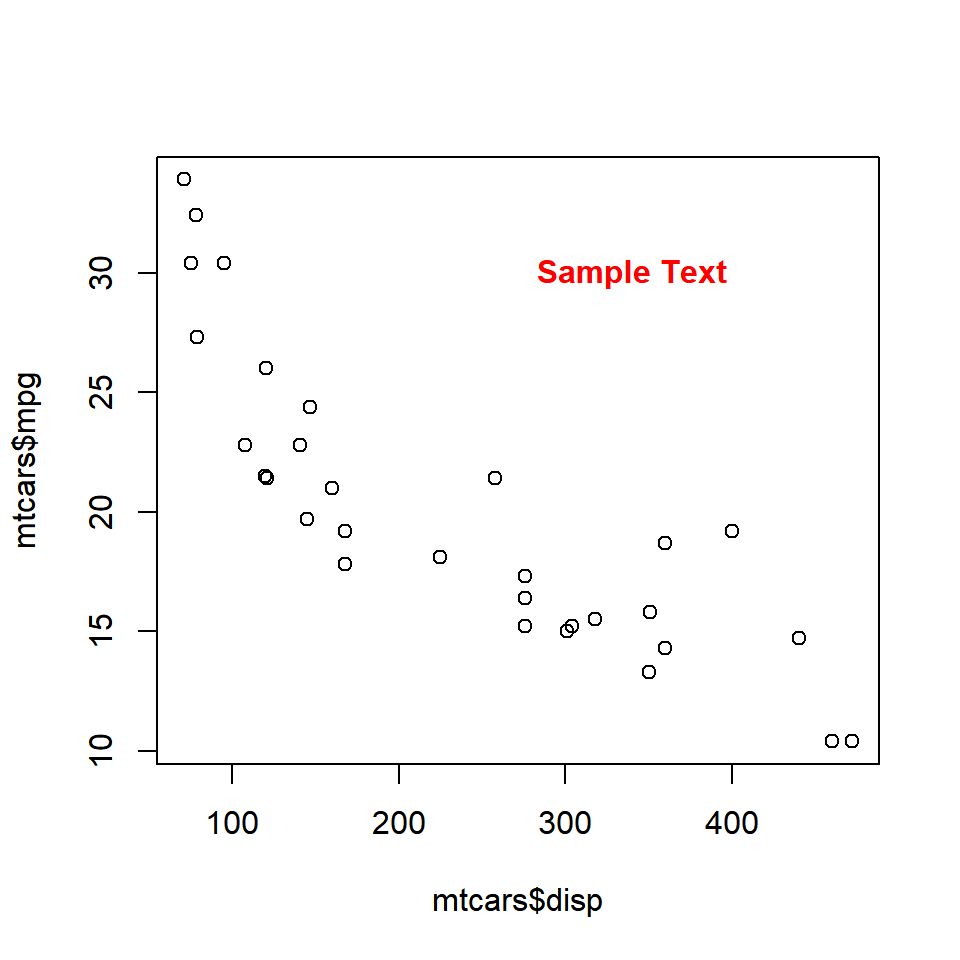The below plot depicts the appearance of the text for different values of the `font` argument:## 9.6 Font Family

The font family of the text can be modified using the `family` argument in the `text()` function.

``````plot(mtcars\$disp, mtcars\$mpg)
text(x = 340, y = 30, labels = 'Sample Text', col = 'red', family = 'mono')``````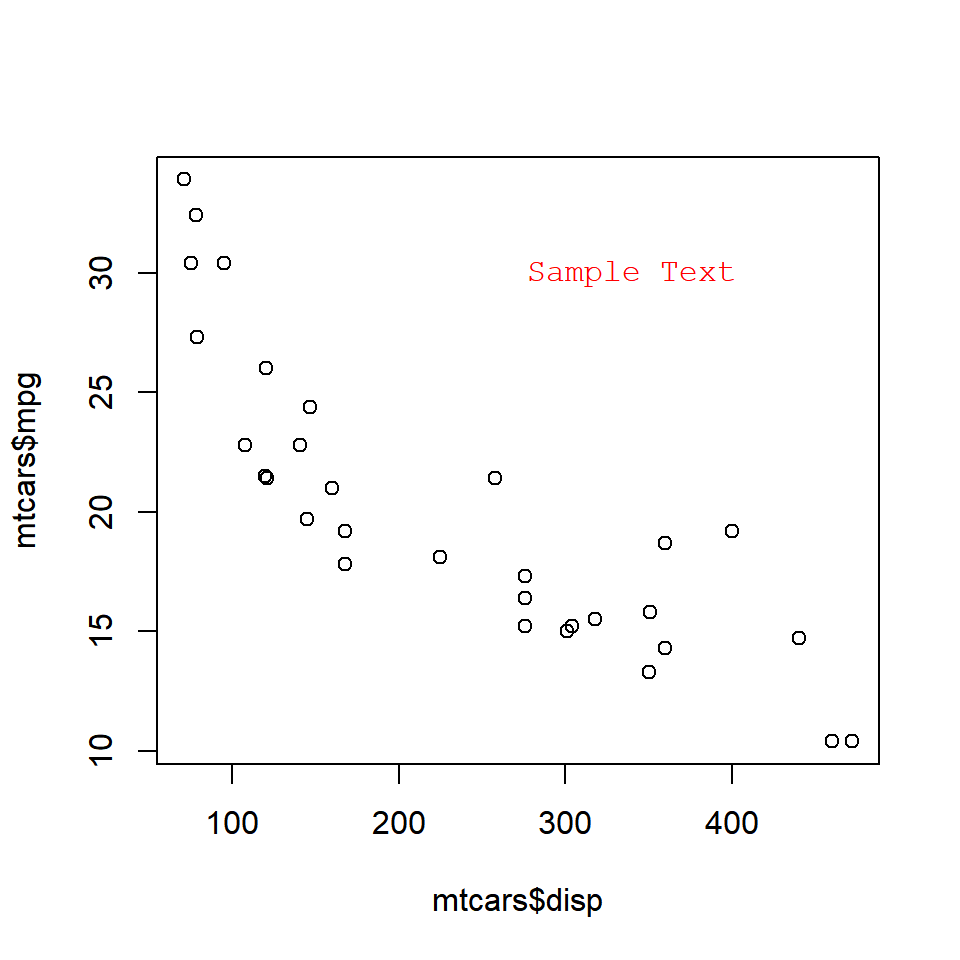The below plot depicts the appearance of the text for different values of the `family` argument:

``````## Warning in text.default(x = 340, y = 30, labels = "Sample Text", col = "red", :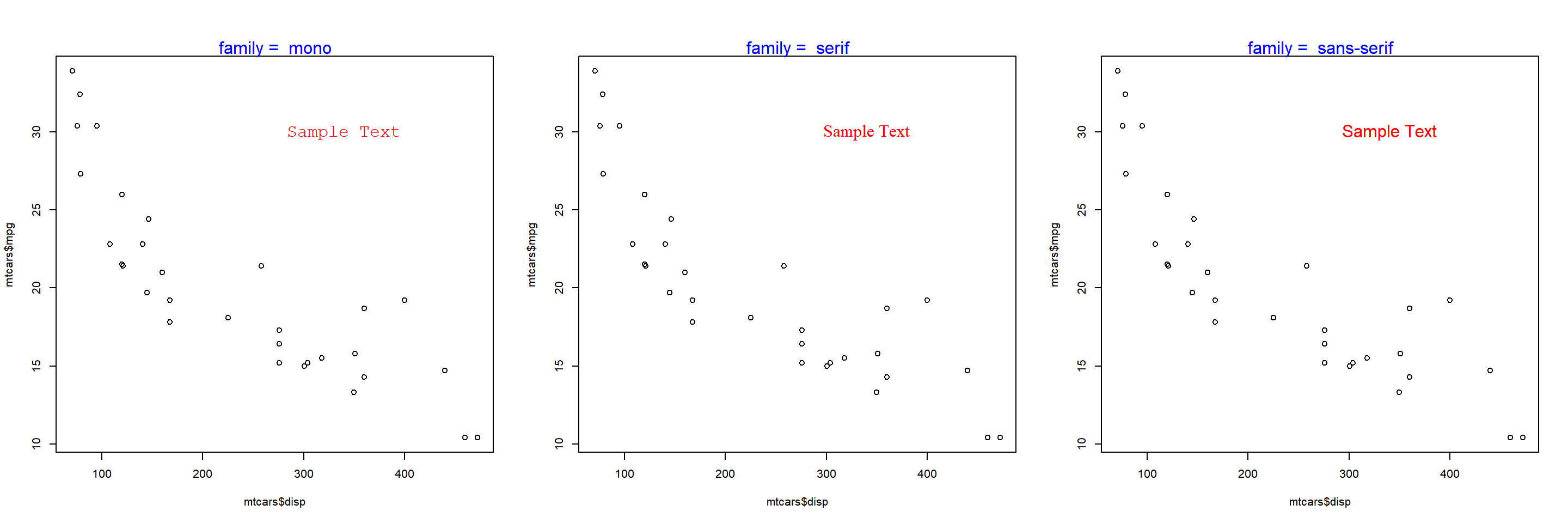## 9.7 Font Size

The font size of the text can be modified using the `cex` argument in the `text()` function.

``````plot(mtcars\$disp, mtcars\$mpg)
text(x = 340, y = 30, labels = 'Sample Text', col = 'red', cex = 2)``````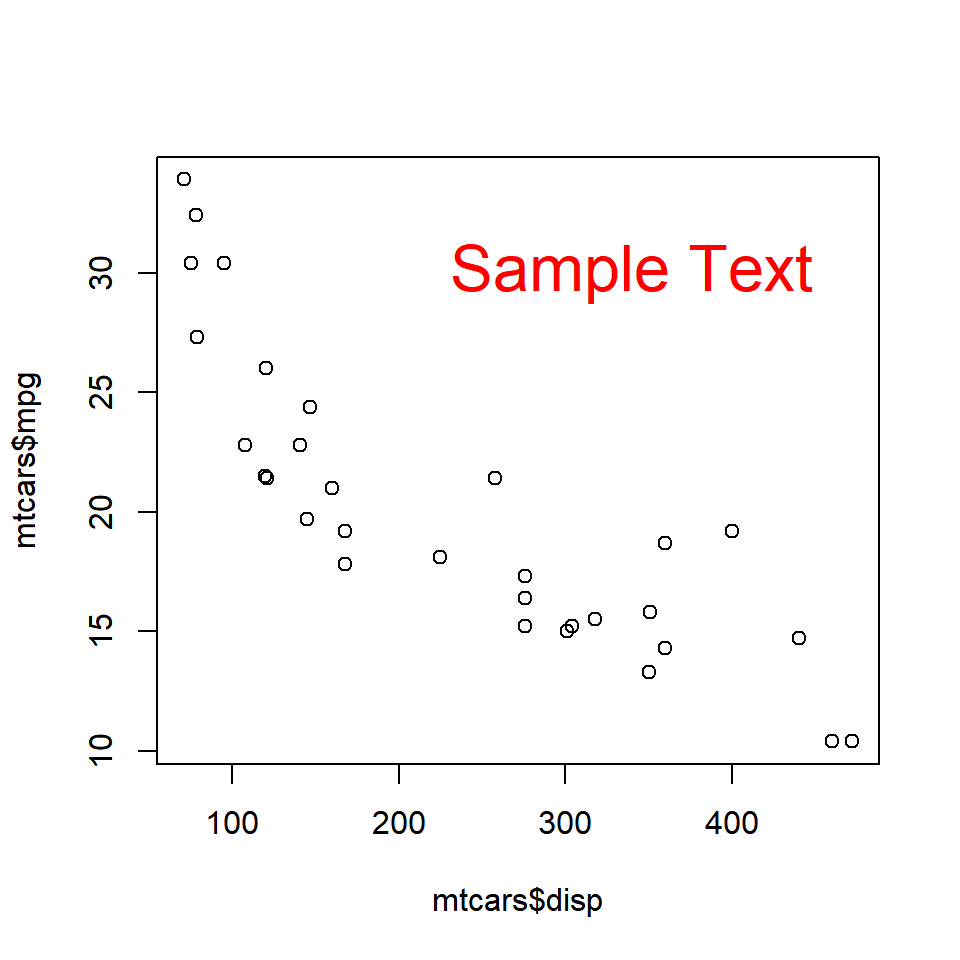The below plot depicts the appearance of the text for different values of the `cex` argument: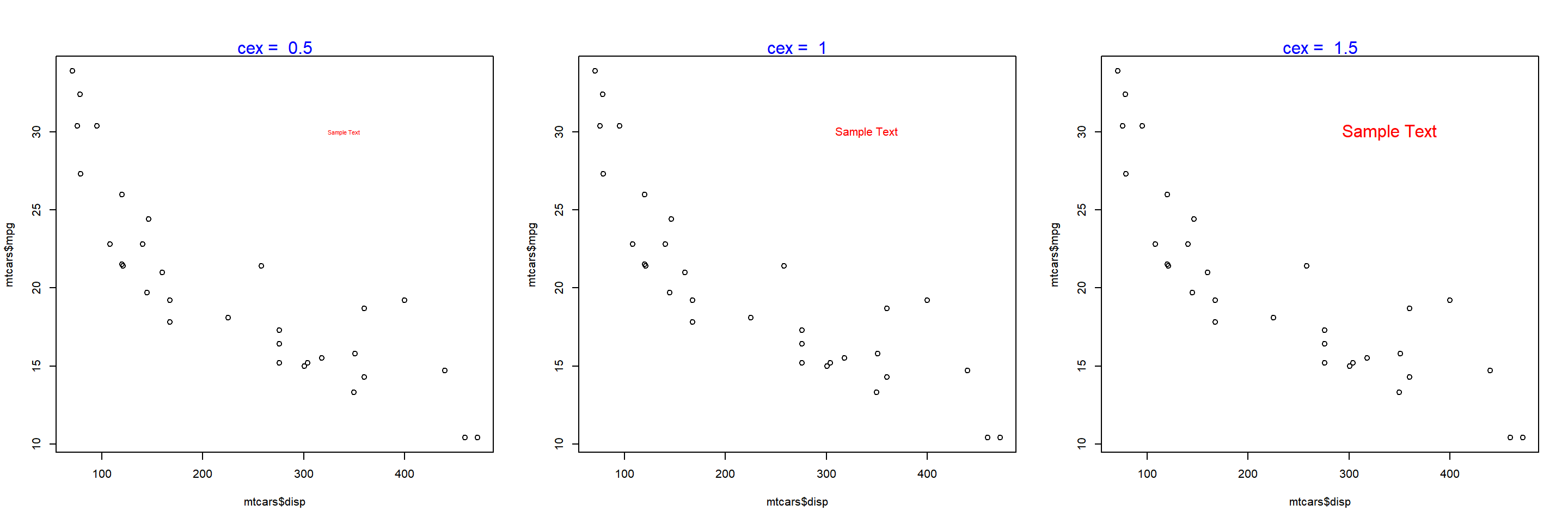## 9.8 Text on the Margins

The `mtext()` function allows the user to place the text on the margins of the plot. It allows the user to modify the location of the text in multiple ways and we will explore them one by one. To beign with, let us add text to the plot using the `mtext()` function. The minimum input you need to provide is the text itself. Below is a simple example:

``````plot(mtcars\$disp, mtcars\$mpg)
mtext('Sample Text')``````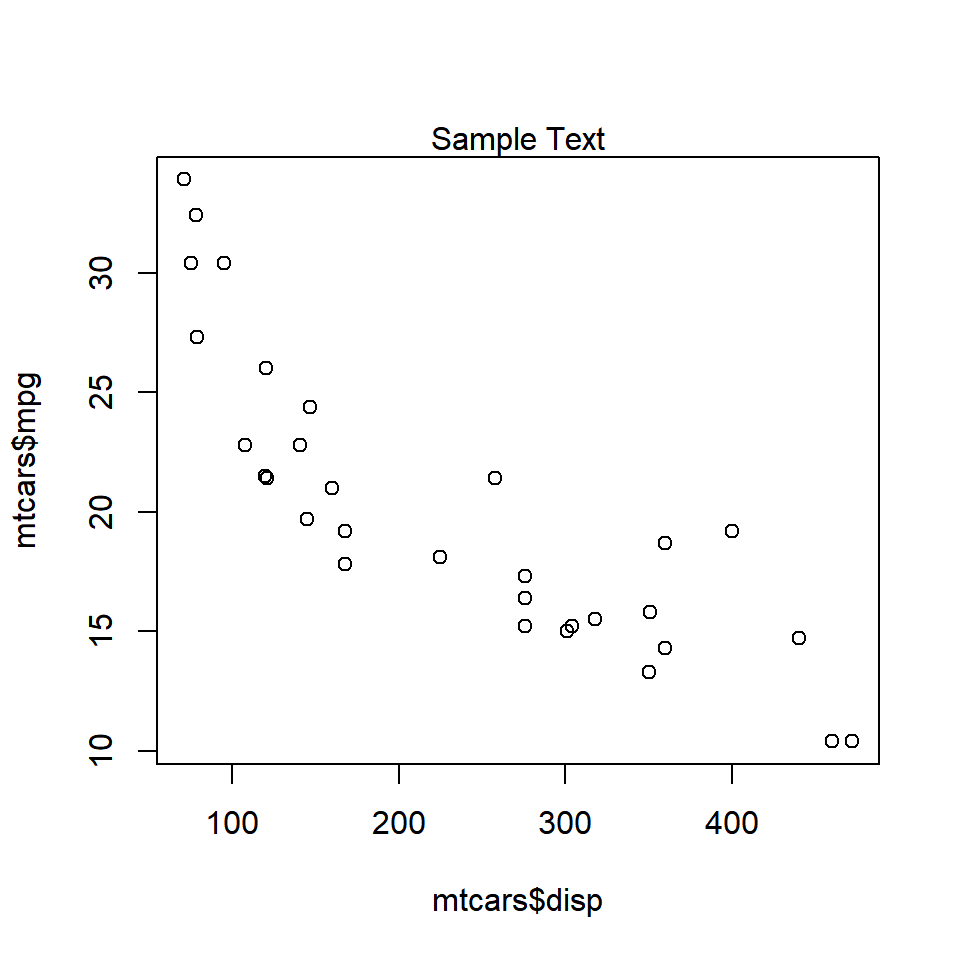As you can see, the text is placed on the margin of the plot and not inside the plot. Next, we will specify the margin on which to place the text.

## 9.9 Specify Margin

Use the `side` argument to specify the margin on which you want to place the text. If takes values 1 to 4, each representing one side of the plot.

``````plot(mtcars\$disp, mtcars\$mpg)
mtext('Sample Text', side = 1)``````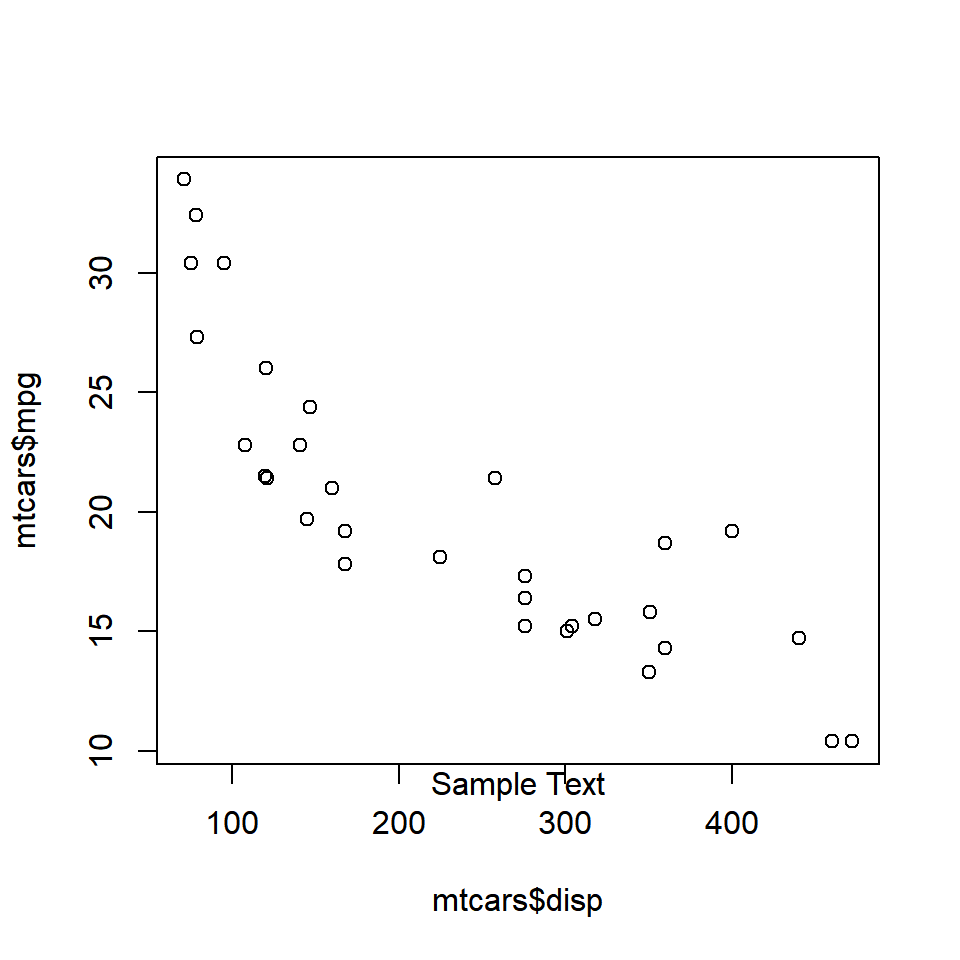The below plot displays the appearance of the text when differnt options for `side` argument are supplied: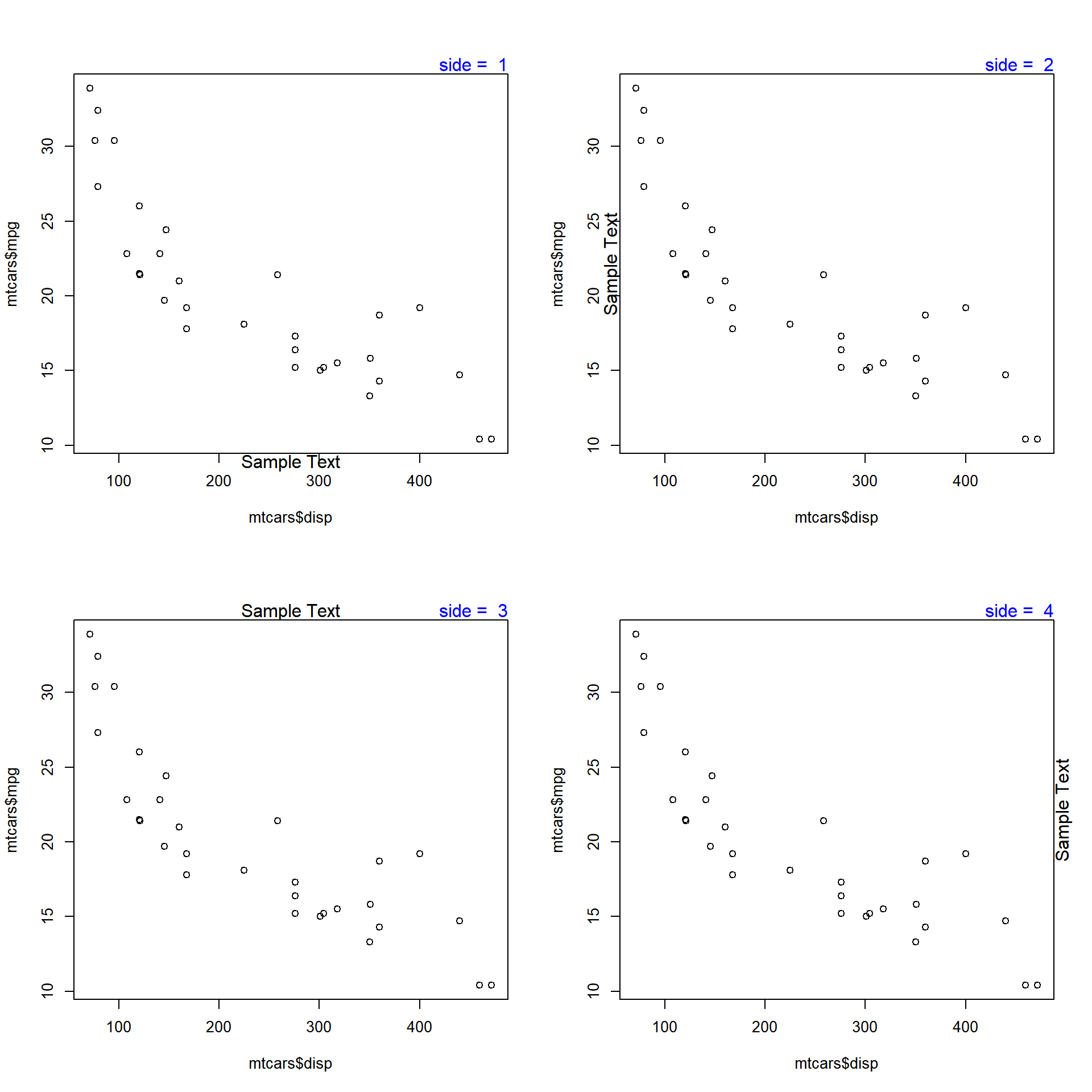## 9.10 Line

The `line` argument places the text at a specified distance from the margin. The default value is `0`. As the value increases, the text is placed farther from the margin and outside the plot. As the value decreases, the text is placed inside the plot and farther from the margin. Below is a example where the text is placed outside the plot as the value is greater than 1.

``````plot(mtcars\$disp, mtcars\$mpg)
mtext('Sample Text', line = 1)``````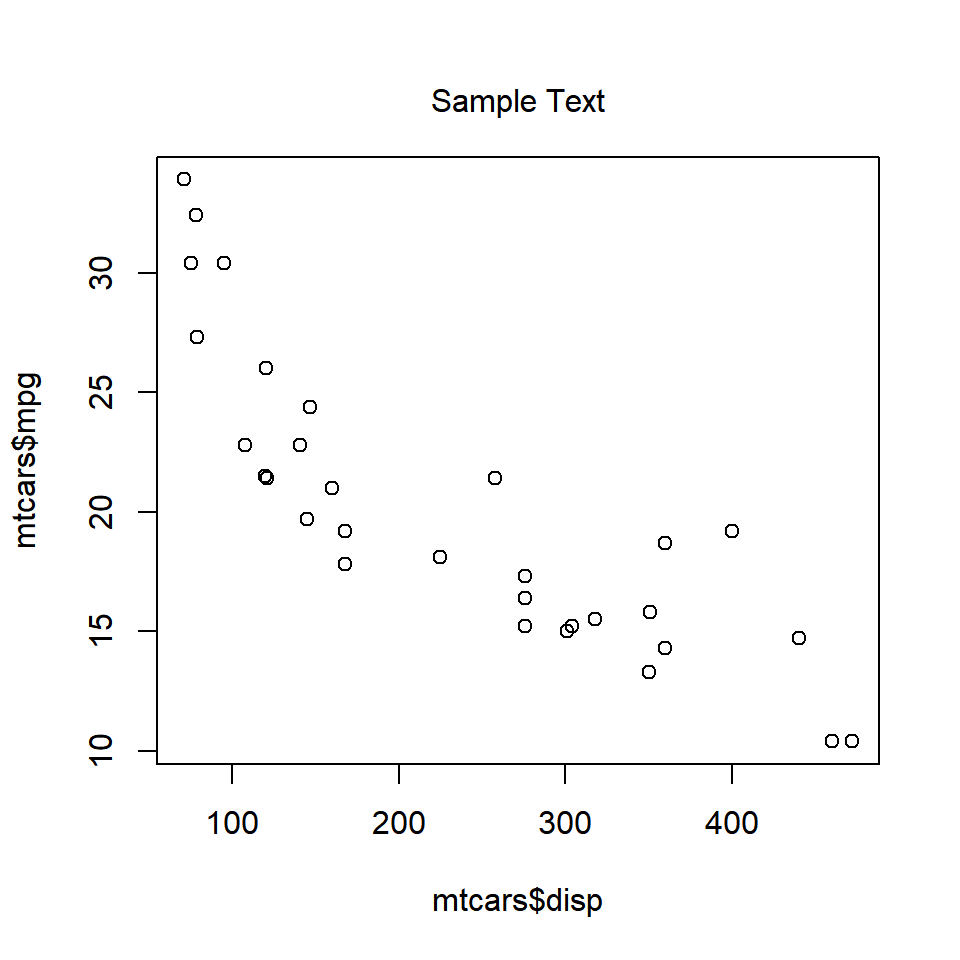When the value is less than `0`, the line argument places the text inside the plot.

``````plot(mtcars\$disp, mtcars\$mpg)
mtext('Sample Text', line = -1)``````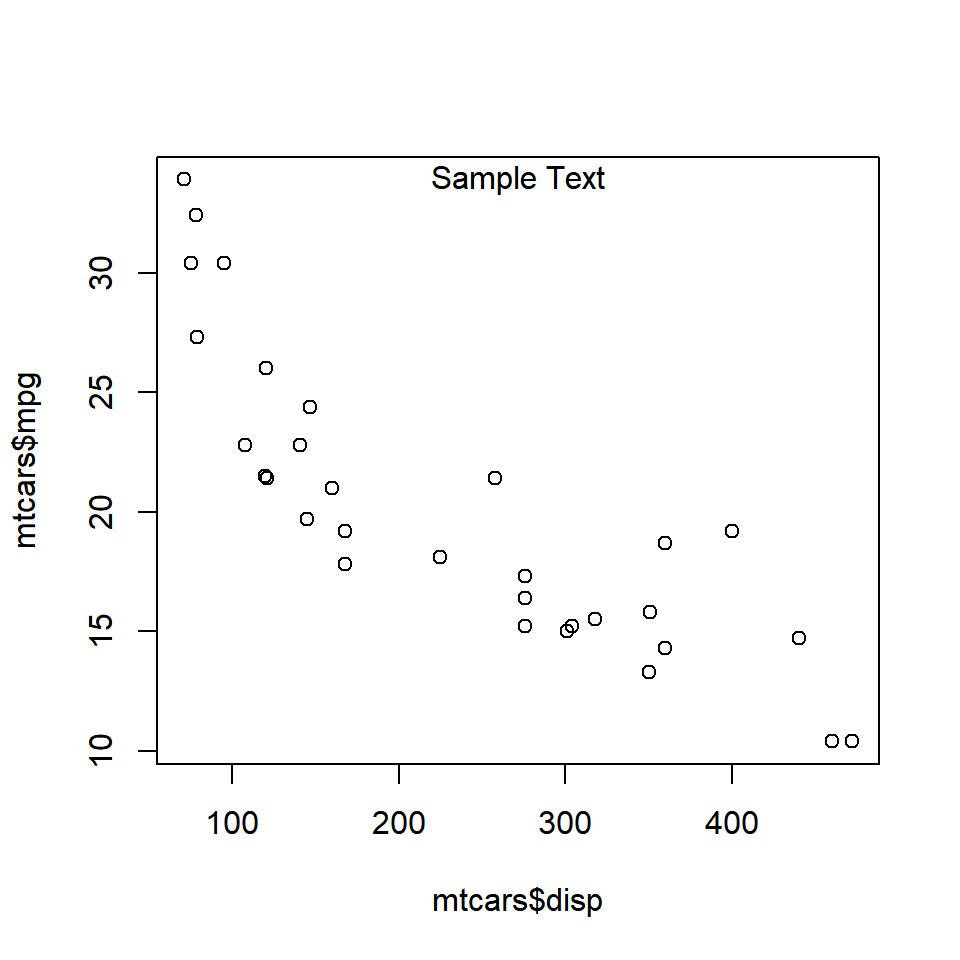The below plot displays the appearance of the text when different values are supplied to the `line` argument: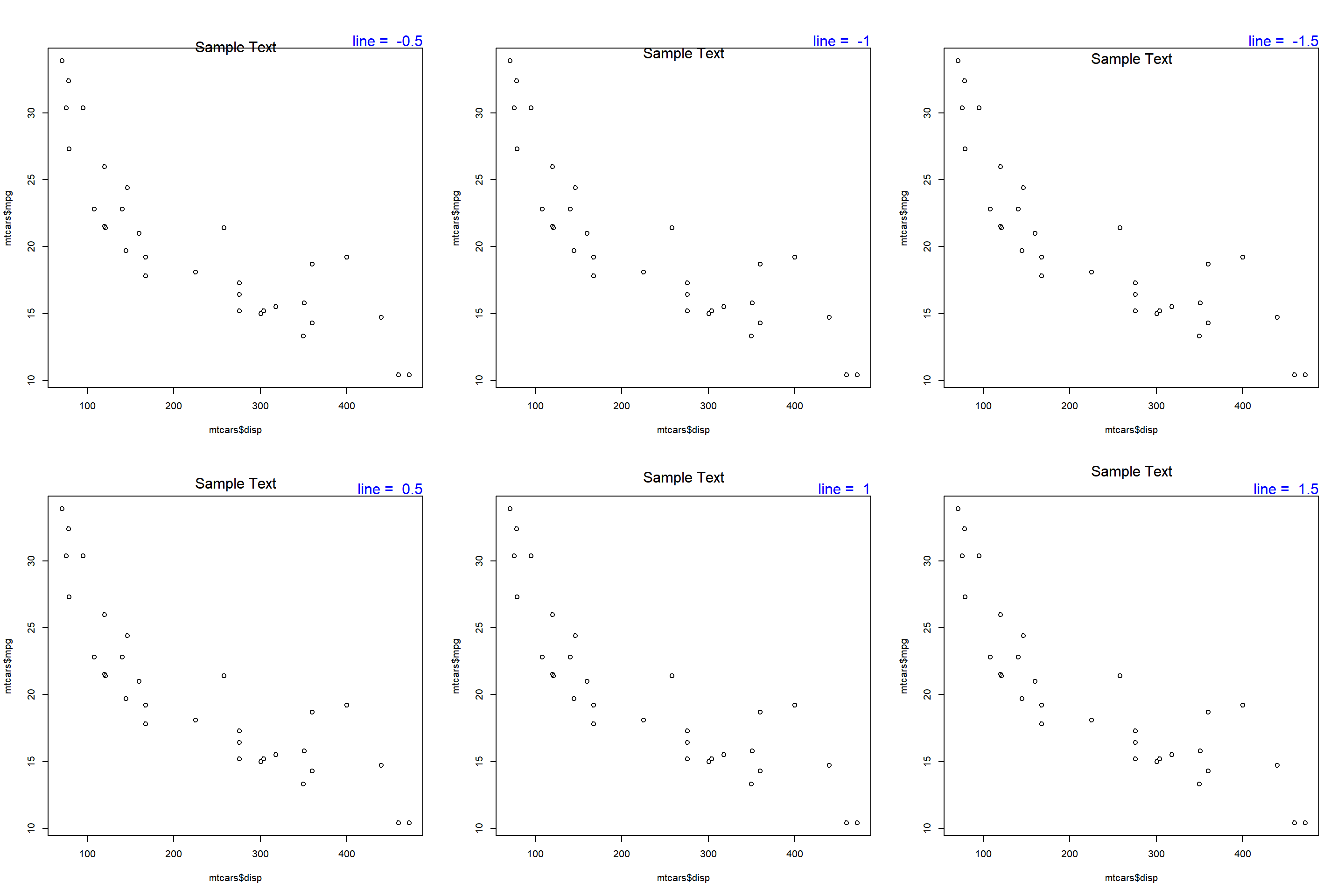## 9.11 Alignment

The `adj` argument is used for horizontal alignment of the text. It takes values between 0 and 1. If set to `0`, the text will be left aligned and at `1`, it will be right aligned. Below is a example where the text is left aligned as `adj` is set to `0`.

``````plot(mtcars\$disp, mtcars\$mpg)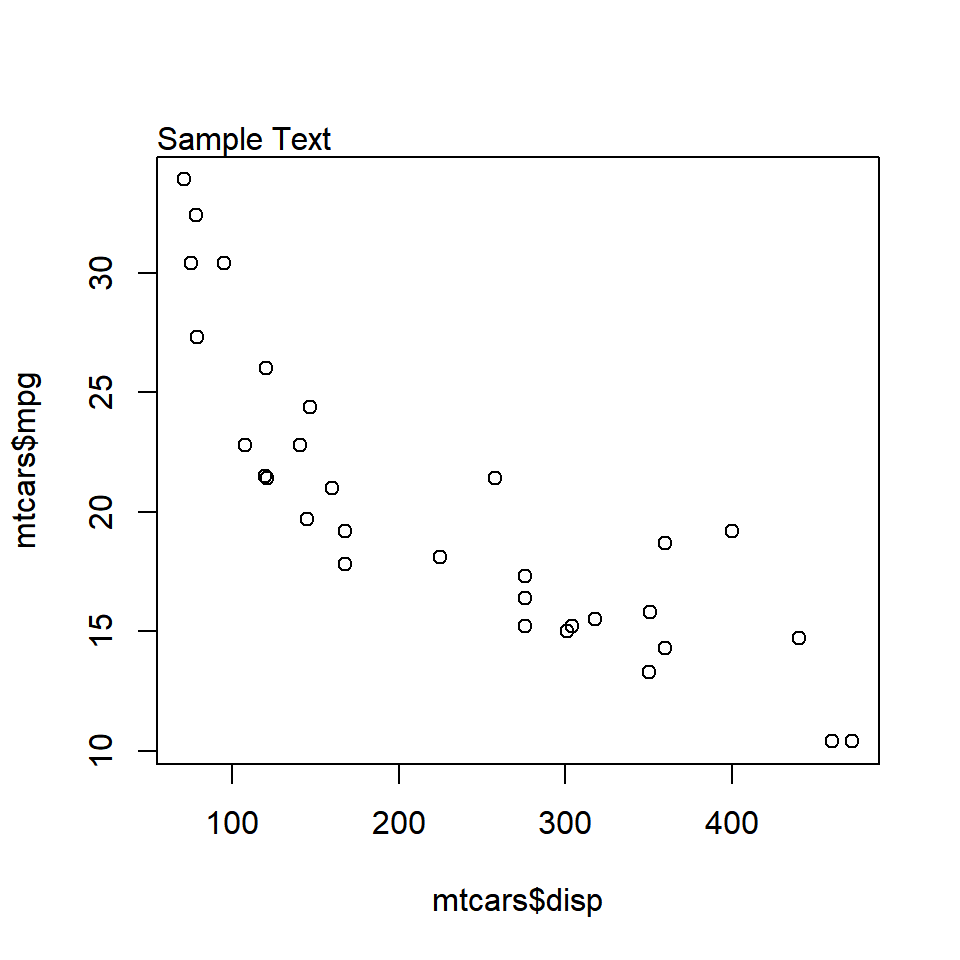When the value is set to `1`, the text is right aligned.
``````plot(mtcars\$disp, mtcars\$mpg)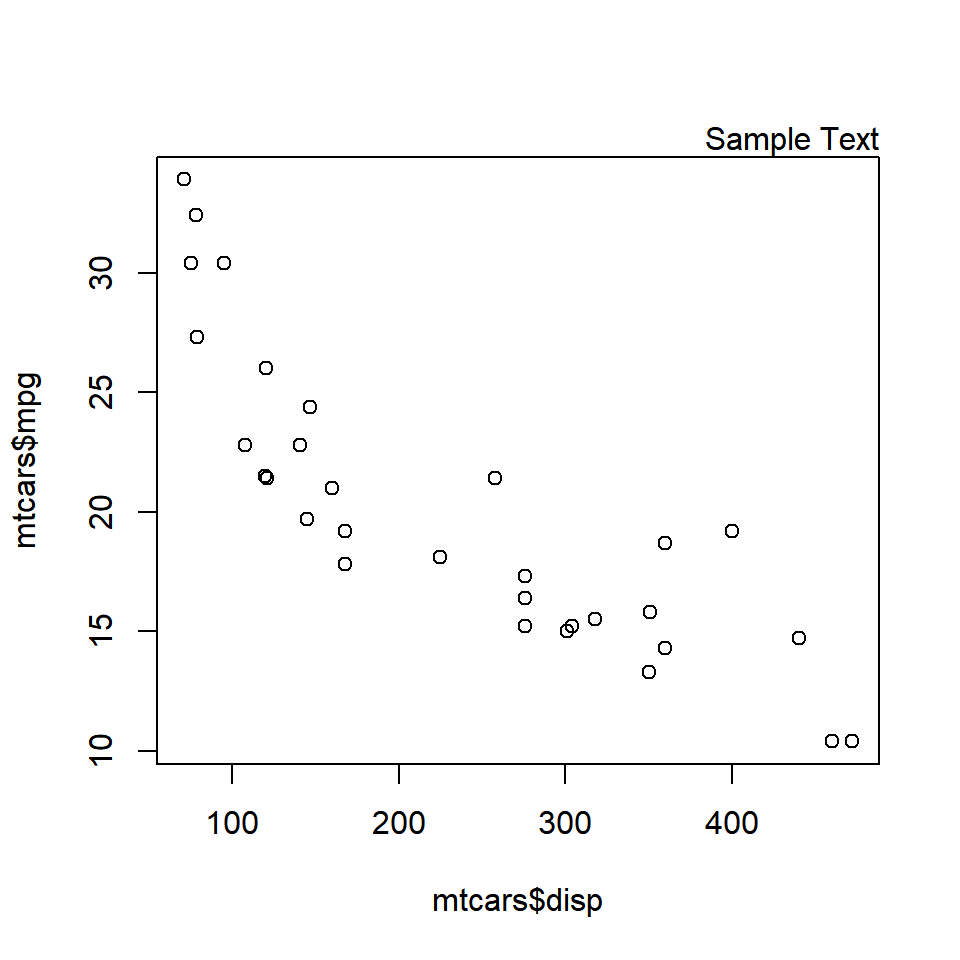The below plot displays the appearance of the text when different values are supplied to the `adj` argument: